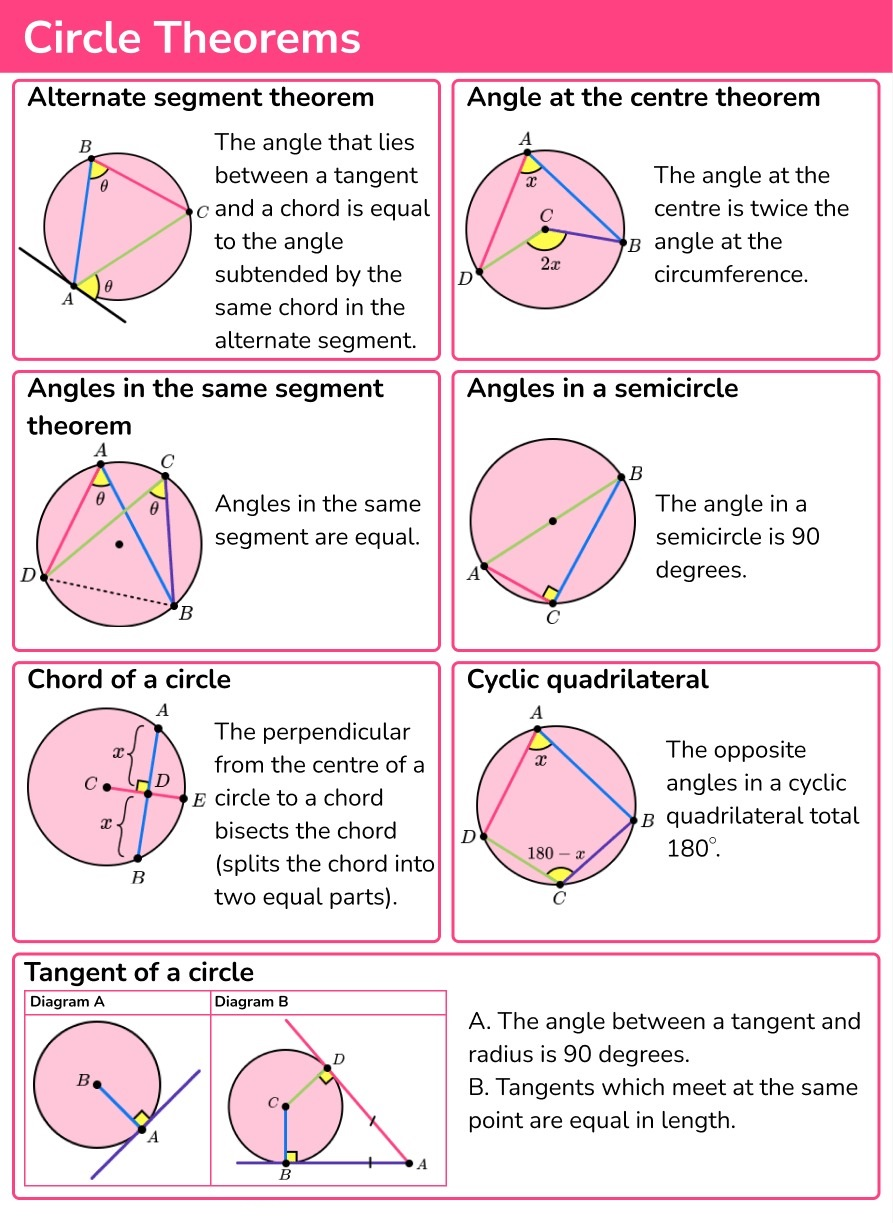### Circle Theorem: Online IGCSE Math Class

Important theorems for circle. The properties of processes provide an understanding of angles generated in the coils. Here are the 8 theorems

• Alternate segment circle theorem.
• The angle at the centre circle theorem.
• Angles in the same segment circle theorem.
• Angle in a semi-circle theorem.
• Chord circle theorem.
• Tangent circle theorem.